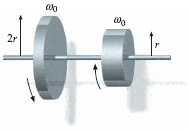# Conservation of angular momentum / finding change in rotational kinetic energy

• ezperkins
I'm not sure where I went wrong. I'll try again later and let you know what happens. Thanks for your help!In summary, the two disks of identical mass but different radii eventually reach a common angular velocity due to the frictional force between the surfaces. The change in rotational kinetic energy is 9/50 of the initial kinetic energy.f

#### ezperkins

Two disks of identical mass but different radii (r and 2r) are spinning on frictionless bearings at the same angular speed ωo, but in opposite directions. The two disks are brought slowly together. The resulting frictional force between the surfaces eventually brings them to a common angular velocity.(a) What is the magnitude of that final angular velocity in terms of ωo
$$\frac{3}{5}$$ωo < - I know that's right.

(b) What is the change in rotational kinetic energy of the system? (Take K as the initial kinetic energy.) This is what I need help with.

I used Ke=Iω2, coupled the system, considered one direction negative and one positive, and got $$\frac{9}{50}$$mr2ωo2, which is apparently wrong. . .

One mistake you made is that the kinetic energy is equal to K=(1/2)Iω2, not Iω2. If that alone isn't the problem, show the details of your calculation so we can see where you might have gone wrong.

Two disks of identical mass but different radii (r and 2r) are spinning on frictionless bearings at the same angular speed ωo, but in opposite directions. The two disks are brought slowly together. The resulting frictional force between the surfaces eventually brings them to a common angular velocity.(a) What is the magnitude of that final angular velocity in terms of ωo
$$\frac{3}{5}$$ωo < - I know that's right.

(b) What is the change in rotational kinetic energy of the system? (Take K as the initial kinetic energy.) This is what I need help with.

I used Ke=Iω2, coupled the system, considered one direction negative and one positive, and got $$\frac{9}{50}$$mr2ωo2, which is apparently wrong. . .

Well, I tried it quite a few different ways, that was just the last wrong answer I got before deciding to ask for help. Here's what I did:

1) $$\Delta$$Ke = Kf - Ki

2) $$\Delta$$Ke = $$\frac{1}{2}$$If$$\omega$$f2 - $$\frac{1}{2}$$Ii$$\omega$$o2

3) $$\frac{1}{2}$$((2m)(3r2))$$\omega$$f2 - $$\frac{1}{2}$$((2m)(3r2))$$\omega$$f2

4) $$\frac{1}{2}$$(6mr2)$$\omega$$f2 - $$\frac{1}{2}$$(6mr2)$$\omega$$o2

5) 3mr2$$\frac{3}{5}$$$$\omega$$o2 - 3mr2$$\omega$$o2

et cetera

I guess I missed some steps the first way through, but I'm sure it's wrong just the same.

I don't follow how you calculated the moment of inertia. Could you explain that?

Perhaps I can just start you out on the right path.First off, the moment of inertia of a disk is

$$I _{\mbox{disk}} = \frac{mr^2}{2}$$

From there, I suggest that you write out the individual initial kinetic energies of each disk, at least as a start. You can always simplify later.

$$K_i = \frac{1}{2}I_1 \omega_0^2 + \frac{1}{2}I_2 \omega_0^2$$

Once you have this written out (as above) you can then simplify it a little now, or simplify it later.

Either way, note that

$$I_1 = \frac{mr^2}{2}$$

and

$$I_2 = \frac{m(2r)^2}{2}$$

Adding these together does not give the ((2m)(3r2)) that you were using in your previous post.

I know how to calculate the moment of inertia. What I don't get is what you did.

Perhaps I can just start you out on the right path.First off, the moment of inertia of a disk is

$$I _{\mbox{disk}} = \frac{mr^2}{2}$$

From there, I suggest that you write out the individual initial kinetic energies of each disk, at least as a start. You can always simplify later.

$$K_i = \frac{1}{2}I_1 \omega_0^2 + \frac{1}{2}I_2 \omega_0^2$$

Once you have this written out (as above) you can then simplify it a little now, or simplify it later.

Either way, note that

$$I_1 = \frac{mr^2}{2}$$

and

$$I_2 = \frac{m(2r)^2}{2}$$

Adding these together does not give the ((2m)(3r2)) that you were using in your previous post.

so $$K_i = \frac{3}{4}mr^2\omega_0^2$$ ?
and $$K_f = \frac{9}{50}mr^2\omega_0^2$$
thus $$\Delta K = \frac{57}{100}mr^2\omega_0^2}$$

I feel like such an idiot. . .

I know how to calculate the moment of inertia. What I don't get is what you did.

Oh, I'm sorry. I basically treated the disks as one big disk.

I have spent more than two and a half hours on this problem and have gotten nowhere. I give up. Thank you vela and collinsmark for your help.

I have spent more than two and a half hours on this problem and have gotten nowhere. I give up. Thank you vela and collinsmark for your help.
You're really close; you're just not calculating the rotational masses correctly, probably just algebra mistakes. Once you clear that up, you'll get the right answer. It might be good to take a break and come back to it later. Errors you can't see right now may pop out clear as day later.

You're really close; you're just not calculating the rotational masses correctly, probably just algebra mistakes. Once you clear that up, you'll get the right answer. It might be good to take a break and come back to it later. Errors you can't see right now may pop out clear as day later.

I tried that already, on Monday and Tuesday.

I guess I'm just not "physics material."

I really appreciate your time though.